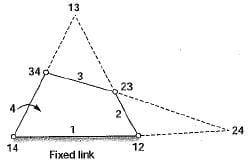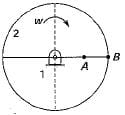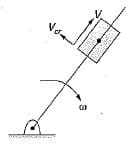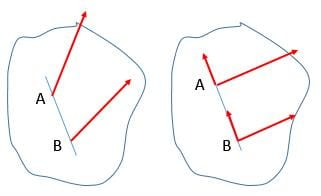Test: Velocity & Acceleration Analysis - 2

# Test: Velocity & Acceleration Analysis - 2

Test Description

## 10 Questions MCQ Test Topicwise Question Bank for Mechanical Engineering | Test: Velocity & Acceleration Analysis - 2

Test: Velocity & Acceleration Analysis - 2 for Mechanical Engineering 2023 is part of Topicwise Question Bank for Mechanical Engineering preparation. The Test: Velocity & Acceleration Analysis - 2 questions and answers have been prepared according to the Mechanical Engineering exam syllabus.The Test: Velocity & Acceleration Analysis - 2 MCQs are made for Mechanical Engineering 2023 Exam. Find important definitions, questions, notes, meanings, examples, exercises, MCQs and online tests for Test: Velocity & Acceleration Analysis - 2 below.
Solutions of Test: Velocity & Acceleration Analysis - 2 questions in English are available as part of our Topicwise Question Bank for Mechanical Engineering for Mechanical Engineering & Test: Velocity & Acceleration Analysis - 2 solutions in Hindi for Topicwise Question Bank for Mechanical Engineering course. Download more important topics, notes, lectures and mock test series for Mechanical Engineering Exam by signing up for free. Attempt Test: Velocity & Acceleration Analysis - 2 | 10 questions in 30 minutes | Mock test for Mechanical Engineering preparation | Free important questions MCQ to study Topicwise Question Bank for Mechanical Engineering for Mechanical Engineering Exam | Download free PDF with solutions
 1 Crore+ students have signed up on EduRev. Have you?
Test: Velocity & Acceleration Analysis - 2 - Question 1

### The Coriolis acceleration component is taken into account for

Test: Velocity & Acceleration Analysis - 2 - Question 2

### The Coriolis acceleration component

Test: Velocity & Acceleration Analysis - 2 - Question 3

### Consider a pin jointed 4-bar mechanism as shown in the figure below.Which of the following are permanent instantaneous centers?

Detailed Solution for Test: Velocity & Acceleration Analysis - 2 - Question 3

I12 and I14 are fixed instantaneous center.
I23 and I34 are permanent instantaneous center.
I13 and I24 are movable instantaneous center.

Test: Velocity & Acceleration Analysis - 2 - Question 4

A crank of radius 15 cm is rotating at 50 rpm with an angular acceleration of 60 rad/sec2. The tangential acceleration of the crank is about

Detailed Solution for Test: Velocity & Acceleration Analysis - 2 - Question 4

at = αr = 60 x 0.15 - 9 m/s

Test: Velocity & Acceleration Analysis - 2 - Question 5

Two points A and B located along the radius of wheel as shown below, have velocities of 80 and 140 m/s respectively.​The distance between the points A and B is 300 mm. The radius of the wheel (in mm) is

Test: Velocity & Acceleration Analysis - 2 - Question 6

The magnitude of the Coriolis component of acceleration of a slider moving at a velocity V on a link rotating at angular speed ω is

Detailed Solution for Test: Velocity & Acceleration Analysis - 2 - Question 6

Magnitude of Coriolis component = 2 \/ω

Test: Velocity & Acceleration Analysis - 2 - Question 7

Klein’s construction can be used when

Detailed Solution for Test: Velocity & Acceleration Analysis - 2 - Question 7

Klein’s construction is used to determine the acceleration of various parts and this method is used when crank has uniform angular velocity.

Test: Velocity & Acceleration Analysis - 2 - Question 8

Coriolis component of acceleration is always

Detailed Solution for Test: Velocity & Acceleration Analysis - 2 - Question 8Coriolis component of acceleration appears in the direction perpendicular to the link.

Test: Velocity & Acceleration Analysis - 2 - Question 9

A constrained kinematic chain (mechanism) has n number of links. What is the number of instantaneous centres

Test: Velocity & Acceleration Analysis - 2 - Question 10

There are two points P and Q on a planar rigid body. The relative velocity between the two points

Detailed Solution for Test: Velocity & Acceleration Analysis - 2 - Question 10

Given any two points A and B on a rigid body, let line AB be passing through points A and B, then the components of velocity of A and B along the line AB are always equal as the distance between A and B never changes or we can say relative velocity along AB is always zero.

Hence the relative velocity of the points must be perpendicular to the line joining them.## Topicwise Question Bank for Mechanical Engineering

58 videos|314 tests
Information about Test: Velocity & Acceleration Analysis - 2 Page
In this test you can find the Exam questions for Test: Velocity & Acceleration Analysis - 2 solved & explained in the simplest way possible. Besides giving Questions and answers for Test: Velocity & Acceleration Analysis - 2, EduRev gives you an ample number of Online tests for practice

## Topicwise Question Bank for Mechanical Engineering

58 videos|314 tests(Scan QR code)